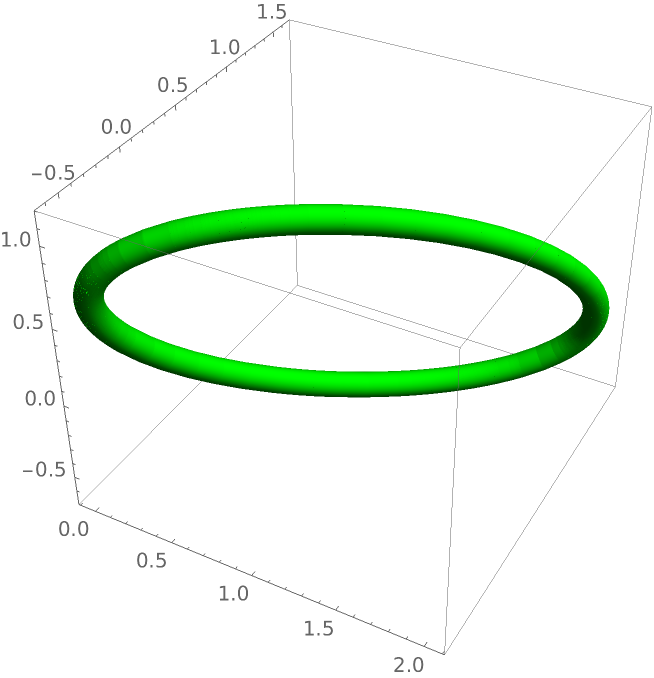Function Repository Resource:

Circle3D

Represent a circle in 3D

Contributed by: Erik Mahieu
 ResourceFunction["Circle3D"][] represents a circe in 3D of radius 1 centered at the origin and perpendicular to the x-axis. ResourceFunction["Circle3D"][{ry,rz}] represents an ellipse in 3D with semiaxes lengths ry and rz centered at the origin and perpendicular to the x-axis. ResourceFunction["Circle3D"][{x,y,z},{ry,rz},ψ,ζ] represents a circe in 3D with semiaxes lengths ry and rz , centerd at {x,y,z} and rotated by angles ψ and ζ around 3D vectors parallel to y- and z-axes respectively.

Details and Options

ResourceFunction["Circle3D"] can be used as a 3D graphics primitive and be used in Graphics3D.
Circle3D takes the Line-primitives generated by the ellipse ParametricPlot3D[{0,a Sin[t],b Cos[t]},{t,-π,π}], translates and rotates them as specified by the function’s parameters: {x,y,z},ψ,ζ.

Examples

Basic Examples

A unit circle in 3D:

 In:=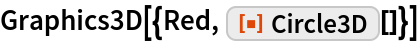Out=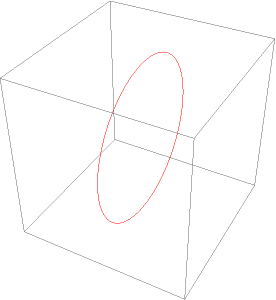An ellipse in 3D:

 In:=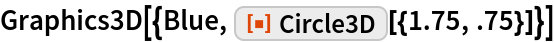Out=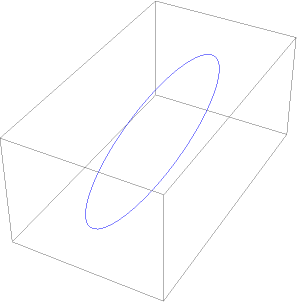Scope

we can use a Manipulate to explore all the parameters of the function Circle3D:

 In:=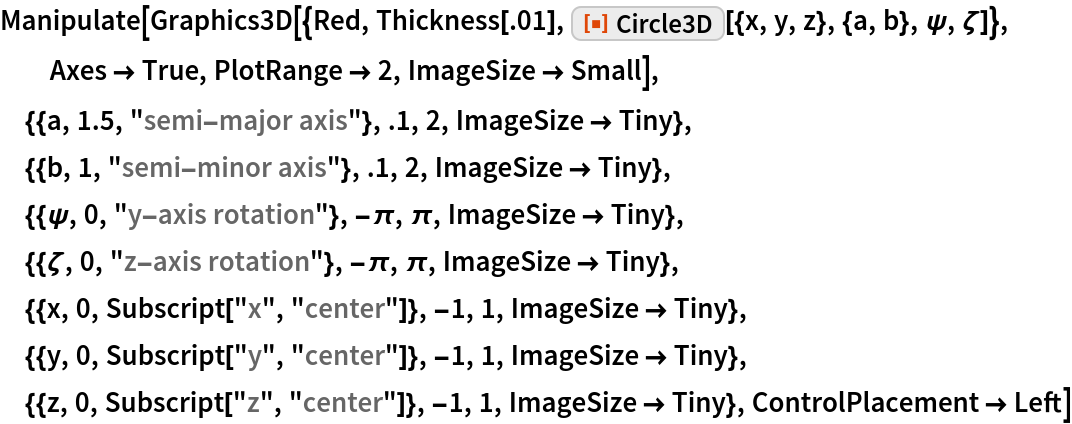Out=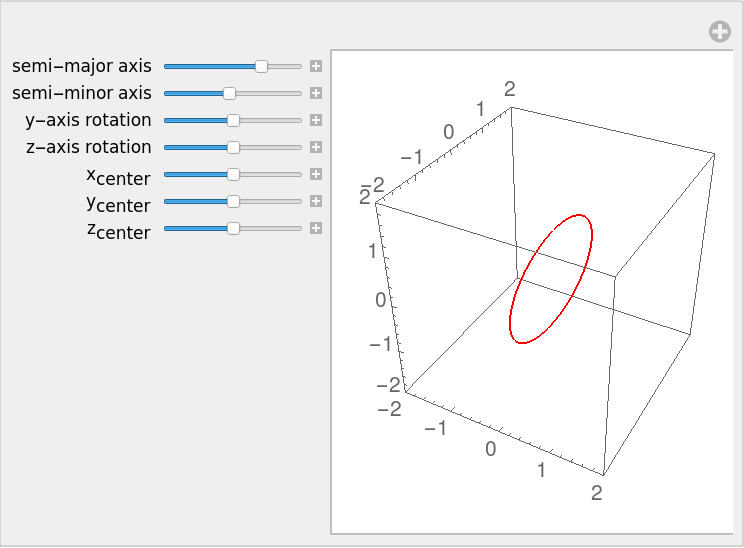Neat Examples

The Line primitives generated by Circle3D can be transformed to Tube:

 In:=Out=